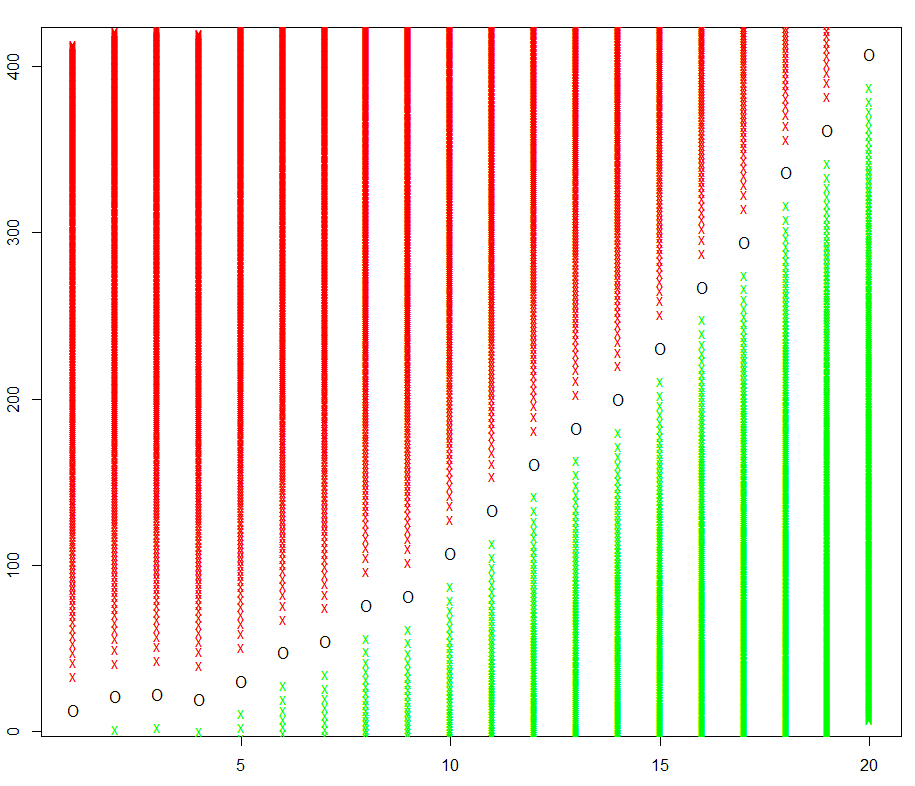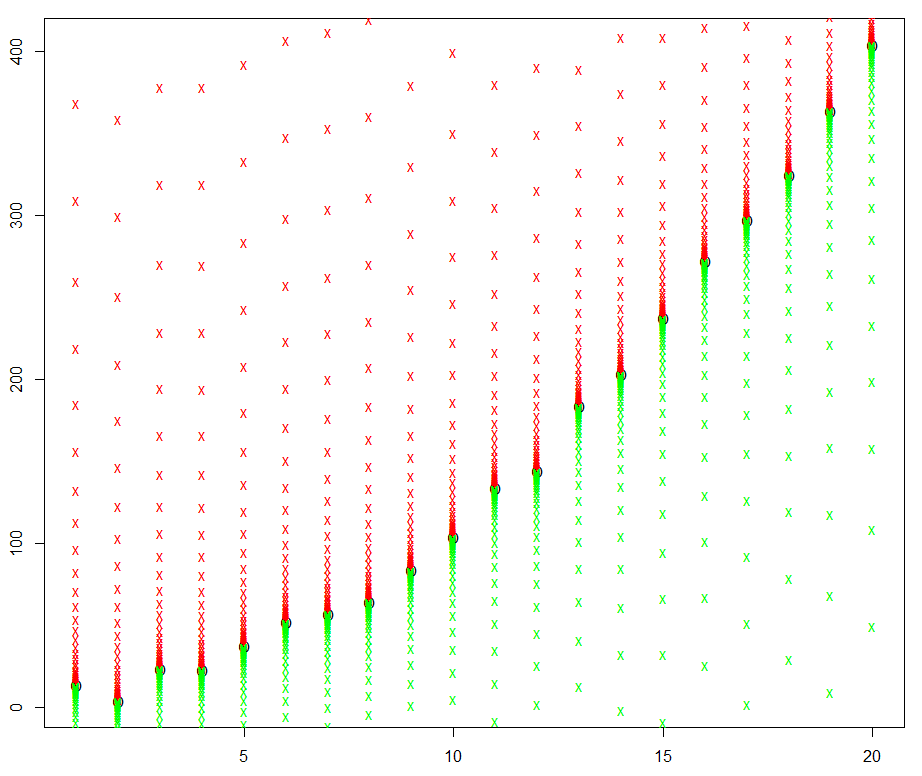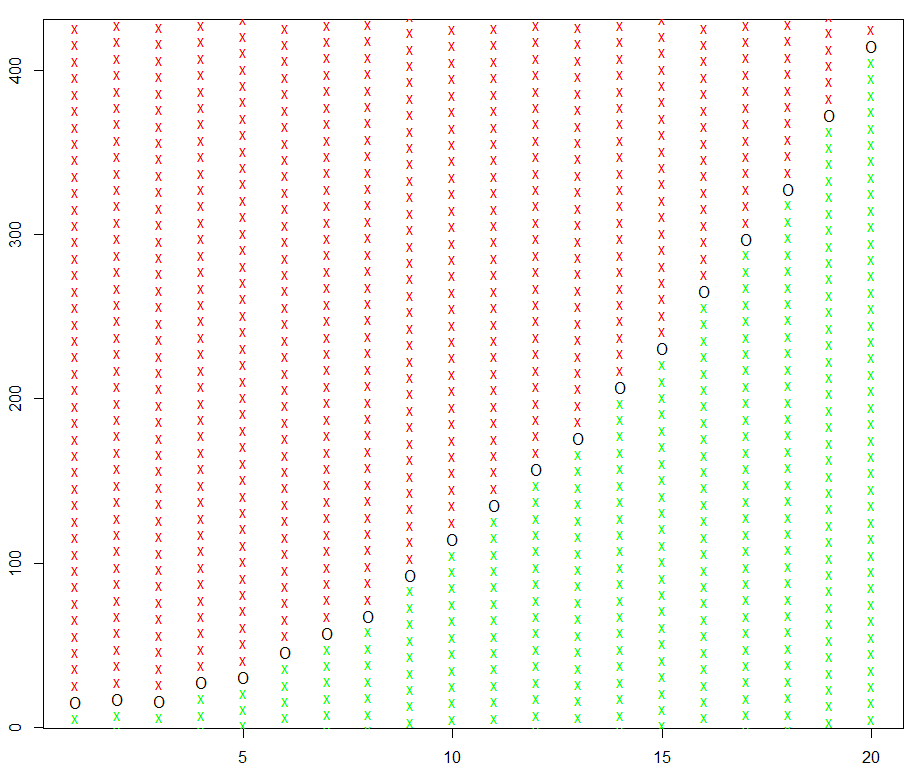LFD Book ForumRegression and Classification Problems
 Register FAQ Calendar Mark Forums Read

#1
 ElrochInvited Guest Join Date: Mar 2013 Posts: 143Regression and Classification Problems

In the course, one interesting thing we looked at was using a tool designed for linear regression to attack classification problems. It proved of some use but less than ideal, for reasons which included the inappropriate cost function (and the inherent awkwardness of approximating a discontinuous step-like function with a linear function). In hindsight this was highly relevant to my own experiments with doing exactly this in the past, if only to see why it was not that great an idea!

But there is a very precise mapping between any regression problem and a classification problem. This mapping is made by including an extra dimension for the domain of the function, and considering the function we are trying to approximate as the boundary of the classification problem.

With this view, every data point we have can be considered to provide an infinite number of data points for classification. If, we knowandfor anyLooking in the other direction, there is a very interesting observation (at least, to me). Suppose we know the value ofmust lie in some interval, then we require one bit of information about f(x) for each halving of this range. Explicitly, we pick the midpoint of the range, classify the point as above or belowand that tells us what we want. We think of functions as infinitely precise but, in the real world, information is finite, and of finite accuracy, and this gives us an explicit relationship between thebits associated with classification ofpoints and a finite precisionof a function.

Anyhow, what I am interested in here is the use of any classification methodology to attack regression problems by this mapping. I am not sure how this relates to, say, the use of SVMs for regression. I imagine it might be efficient to turn each data point for a regression problem into two points for classification, by adding and subtracting a small quantity and considering them to be classified above and below the function surface, but it would likely be useful to have other points to get a better relationship between the error functions. With the above idea in mind, one possibility is to space the points for classification in geometric progression (getting more widely spaced away from the function/boundary), so that classification errors are directly related to the number of bits of accuracy of the resulting regression function! Other spacings of the points can be chosen to correspond to any pointwise error function.

Can anyone clarify how these ideas relate to the current state of knowledge and technology?
#2htlinNTU Join Date: Aug 2009 Location: Taipei, Taiwan Posts: 610Re: Regression and Classification Problems

Quote:
 Originally Posted by ElrochIn the course, one interesting thing we looked at was using a tool designed for linear regression to attack classification problems. It proved of some use but less than ideal, for reasons which included the inappropriate cost function (and the inherent awkwardness of approximating a discontinuous step-like function with a linear function). In hindsight this was highly relevant to my own experiments with doing exactly this in the past, if only to see why it was not that great an idea! But there is a very precise mapping between any regression problem and a classification problem. This mapping is made by including an extra dimension for the domain of the function, and considering the function we are trying to approximate as the boundary of the classification problem. With this view, every data point we have can be considered to provide an infinite number of data points for classification. If, we knowandfor anyLooking in the other direction, there is a very interesting observation (at least, to me). Suppose we know the value ofmust lie in some interval, then we require one bit of information about f(x) for each halving of this range. Explicitly, we pick the midpoint of the range, classify the point as above or belowand that tells us what we want. We think of functions as infinitely precise but, in the real world, information is finite, and of finite accuracy, and this gives us an explicit relationship between thebits associated with classification ofpoints and a finite precisionof a function. Anyhow, what I am interested in here is the use of any classification methodology to attack regression problems by this mapping. I am not sure how this relates to, say, the use of SVMs for regression. I imagine it might be efficient to turn each data point for a regression problem into two points for classification, by adding and subtracting a small quantity and considering them to be classified above and below the function surface, but it would likely be useful to have other points to get a better relationship between the error functions. With the above idea in mind, one possibility is to space the points for classification in geometric progression (getting more widely spaced away from the function/boundary), so that classification errors are directly related to the number of bits of accuracy of the resulting regression function! Other spacings of the points can be chosen to correspond to any pointwise error function. Can anyone clarify how these ideas relate to the current state of knowledge and technology?
Problem 3.13 of the LFD book illustrates an idea similar to yours.

There are works that try to systematically connection regression to classification. The one you see in SVM regression more or less follows from the idea of "loss symmetrization" (You can google for some related work back ten years ago).

For bounded-range regression, there are works like

John Langford and Bianca Zadrozny Estimating Class Membership Probabilities Using Classifier Learners AISTAT 2005

based on using classifiers to decide suitable "thresholds" within the range.

In works that reduce regression to classification, another key issue is usually about whether the reduced problems are "easy enough" to be solved well by classifiers. For instance, classifying every bit of the real-valued target separately may be challenging for classifiers, because you'd essentially need a high-frequency function (i.e. complex classifier) for the low-order bits.

Hope this helps.
__________________
When one teaches, two learn.
#3
 ElrochInvited Guest Join Date: Mar 2013 Posts: 143Re: Regression and Classification Problems

Thanks, Lin (is your last name your given name, in the Chinese style?).

Following the principle that a picture is worth a thousand words, I thought I would post a couple instead of 2000 words.

Here is the classification error equivalent to mean square error regression (with a fairly crude quantisation to make it less painful to look at)and here is the classification error emulation of the wacky but natural "bit error regression" (where the error function is proportional to the complement of the number of correct leading bits in the values).The above option may be entirely useless (although it can be dangerous to guess that), but a less crazy looking example is L1 regression error:Thread ToolsShow Printable VersionEmail this Page Display ModesLinear ModeSwitch to Hybrid ModeSwitch to Threaded ModePosting Rules You may not post new threads You may not post replies You may not post attachments You may not edit your posts BB code is On Smilies are On [IMG] code is On HTML code is Off Forum Rules
 Forum Jump User Control Panel Private Messages Subscriptions Who's Online Search Forums Forums Home General     General Discussion of Machine Learning     Free Additional Material         Dynamic e-Chapters         Dynamic e-Appendices Course Discussions     Online LFD course         General comments on the course         Homework 1         Homework 2         Homework 3         Homework 4         Homework 5         Homework 6         Homework 7         Homework 8         The Final         Create New Homework Problems Book Feedback - Learning From Data     General comments on the book     Chapter 1 - The Learning Problem     Chapter 2 - Training versus Testing     Chapter 3 - The Linear Model     Chapter 4 - Overfitting     Chapter 5 - Three Learning Principles     e-Chapter 6 - Similarity Based Methods     e-Chapter 7 - Neural Networks     e-Chapter 8 - Support Vector Machines     e-Chapter 9 - Learning Aides     Appendix and Notation     e-Appendices

All times are GMT -7. The time now is 08:58 PM.The contents of this forum are to be used ONLY by readers of the Learning From Data book by Yaser S. Abu-Mostafa, Malik Magdon-Ismail, and Hsuan-Tien Lin, and participants in the Learning From Data MOOC by Yaser S. Abu-Mostafa. No part of these contents is to be communicated or made accessible to ANY other person or entity.